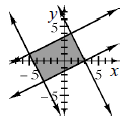### Home > INT3 > Chapter 3 > Lesson 3.2.1 > Problem3-81

3-81.

Make a complete graph of the four inequalities below on the same set of axes.

1. $2y\ge x-3$

1. $x-2y\ge-7$

1. $y\le-2x+6$

1. $-9\le2x+y$1. What type of polygon is formed by the solution of this set of inequalities? Write a convincing argument to justify your answer.

How does the slope of each line compare to the others?

2. What are the vertices of the polygon? If your graph is very accurately drawn, you will be able to determine the points from the graph. If it is not, you will need to solve the systems (pairs) of equations that represent the corners of your graphs.

$(1,4),(−3,−3),(−5,1),(3,0)$